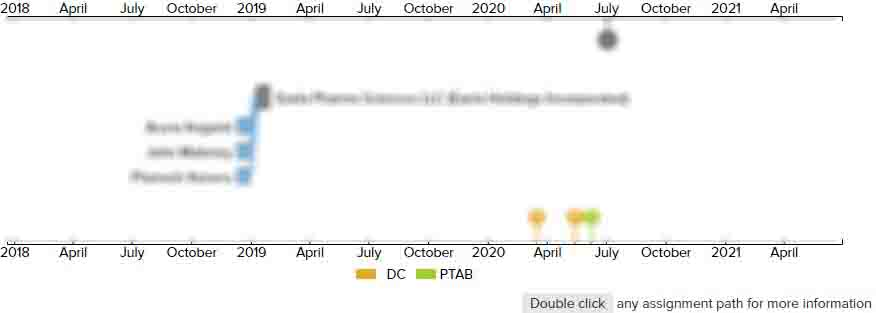# SYSTEMS AND METHODS FOR DETERMINING INVESTMENT STRATEGIES

• US 20110270782A1
• Filed: 04/30/2010
• Published: 11/03/2011
• Est. Priority Date: 04/30/2010
• Status: Abandoned Application
##### First Claim
Patent Images

1. A method performed in a processor system that defines asset portfolio valuations over a study period, wherein the study period is defined by a plurality of sequential incremental periods, the method comprising:

• retrieving an initial asset portfolio defining a plurality of assets and associated asset values from a memory communicatively coupled to the processor system;

performing a plurality of iterations for each of the incremental periods of the study period, wherein each iteration comprises;

retrieving from the memory a base asset return value defined for the incremental period;

determining a plurality of iteration asset return values from a predefined statistical function and the base asset return value, wherein each of the plurality of iteration asset return values represents a possible rate of return of the a value of the plurality of assets of the asset portfolio for the incremental period;

retrieving from the memory a base inflation value defined for the incremental period;

determining a plurality of iteration inflation values from the predefined statistical function and the base inflation value, wherein each of the plurality of iteration inflation values represents a possible rate of inflation affecting the value of the plurality of assets of the asset portfolio for the incremental period;

retrieving from the memory a base tax value defined for the incremental period;

determining a plurality of iteration tax values from the predefined statistical function and the base tax value, wherein each of the plurality of iteration tax values represents a possible tax rate affecting earnings of the plurality of assets of the asset portfolio for the incremental period;

determining an iteration asset portfolio value for the iteration based upon the plurality of iteration asset return values, the plurality of iteration inflation values, the plurality of iteration tax values, and the plurality of asset values of the asset portfolio; and

determining a value of the asset portfolio for each of the incremental periods based upon the iteration asset portfolio values respectively determined for each one of the incremental periods; and

generating a financial report based upon the asset portfolio values determined for each of the incremental periods, wherein the generated financial report indicates changes of the asset portfolio value over the study period.

• ##### 1 Assignment
Timeline View
Assignment View×
×